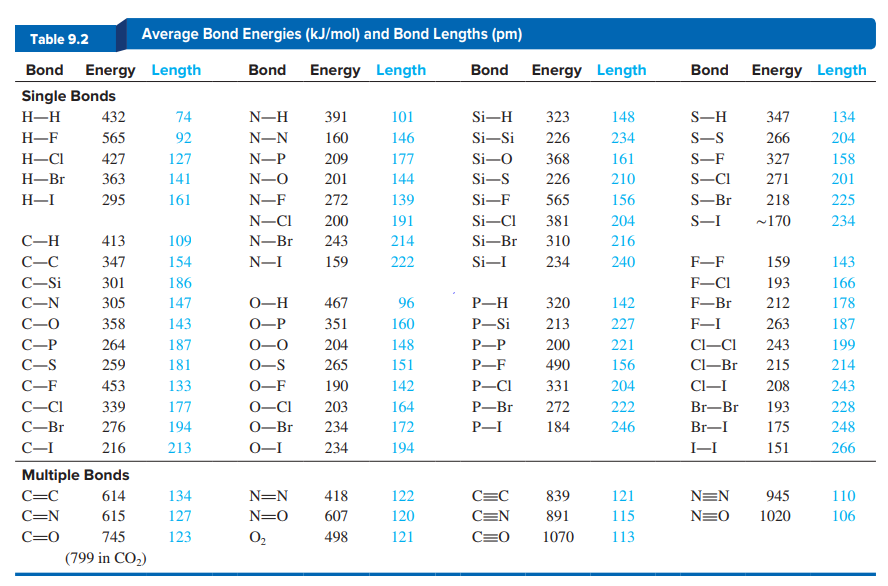Chemistry Practice Problems Bond Angles Practice Problems Solution: Using bond lengths in Table 9.2 and assuming ideal...

⚠️Our tutors found the solution shown to be helpful for the problem you're searching for. We don't have the exact solution yet.

# Solution: Using bond lengths in Table 9.2 and assuming ideal bond angles, calculate each of the following distances:(c) Between equatorial F atoms in PF5

###### Problem

Using bond lengths in Table 9.2 and assuming ideal bond angles, calculate each of the following distances:

(c) Between equatorial F atoms in PF5Bond Angles

Bond Angles

#### Q. Which species has the smaller bond angle, H3O+ or H2O?

Solved • Mon Nov 12 2018 12:41:01 GMT-0500 (EST)

Bond Angles

#### Q. Ethylene, C2H4, and tetrafluoroethylene, C2F4, are used to make the polymers polyethylene and polytetrafluoroethylene (Teflon), respectively.(a) Draw ...

Solved • Sun Oct 28 2018 13:53:25 GMT-0400 (EDT)

Bond Angles

#### Q. Because both tin and carbon are members of Group 4A(14), they form structurally similar compounds. But tin exhibits a greater variety of structures be...

Solved • Sun Oct 28 2018 05:13:36 GMT-0400 (EDT)

Bond Angles

#### Q. Arrange the following ACln species in order of  decreasing  Cl—A—Cl bond angles:SCl2, OCl2, PCl3, SiCl4, SiCl62−.

Solved • Sun Oct 28 2018 05:03:16 GMT-0400 (EDT)